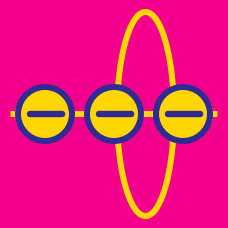Electricity and Magnetism

# Field lines and field strength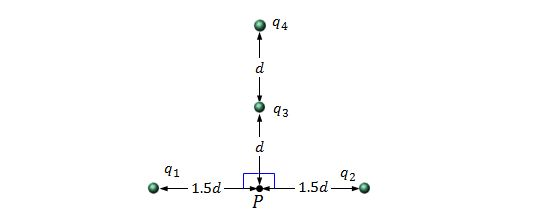Four charged particles are held on the same plane, as shown in the above figure. The respective charges of the particles are $q_1=q_2=+5.0 \,\mu\text{C},$ $q_3=+4.0 \,\mu\text{C},$ and $q_4=-16.0 \,\mu\text{C},$ and the distance $d$ in the figure is $8.0 \text{ mm}.$ What is the magnitude of the net electric field at point $P$ due to the four particles?

The value of electrostatic constant is $\displaystyle k=\frac{1}{4\pi\varepsilon_0}=8.99 \times 10^9 \text{ N}\cdot\text{m}^2\text{/C}^2.$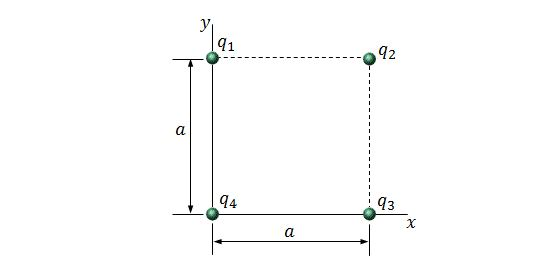Four particles are at the corners of a square with side length $a=5.00\text{ cm},$ as shown in the above figure. The respective charges of the particles are $q_1=+12.0\text{ nC},$ $q_2=-24.0\text{ nC},$ $q_3=+24.0\text{ nC},$ and $q_4=-12.0\text{ nC}.$ What are the magnitude and direction of the net electric field at the center of the square?

The value of electrostatic constant is $\displaystyle k=\frac{1}{4\pi\varepsilon_0}=8.99 \times 10^9 \text{ N}\cdot\text{m}^2\text{/C}^2.$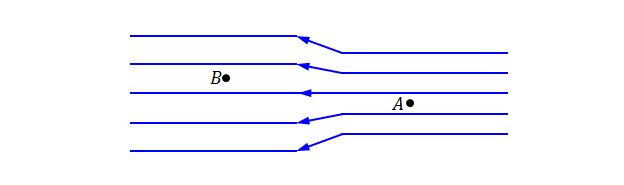In the above figure, the separation of electric field lines on the left is twice the separation of those on the right. If the magnitude of the field at position A is $70\text{ N/C},$ what is the magnitude of the field at position B?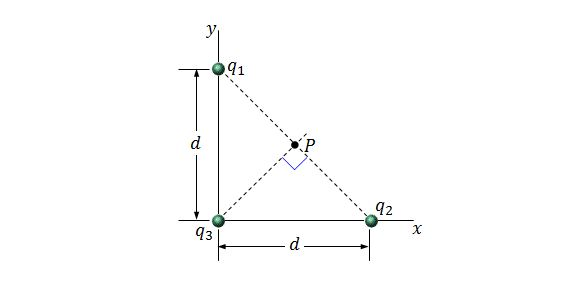Three charged particles are held on the $xy$-plane, as shown in the above figure. The distance denoted $d$ in the figure is $d=6.00\,\mu\text{m}.$ If the respective charges of the particles are $q_1=q_2=+4e$ and $q_3=+8e,$ what is the magnitude of the net electric field at point $P$ due to the three charged particles?

The value of electrostatic constant is $\displaystyle k=\frac{1}{4\pi\varepsilon_0}=8.99 \times 10^9 \text{ N}\cdot\text{m}^2\text{/C}^2$ and the elementary charge is $e=1.60 \times 10^{-19}\text{ C}.$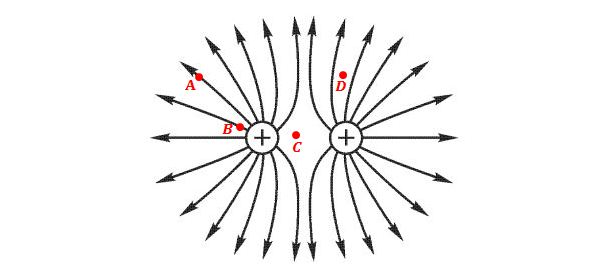The figure above portrays the electric field produced by two positive-charged particles. Which of the following correctly lists the points A, B, C, and D in order of increasing electric field strength?

×